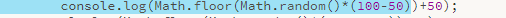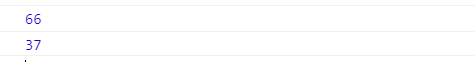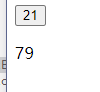• JavaScript有提供一个生成值区间在(0, 1)的随机小数的函数。 Math.random(); // 0.10529863457509858 ...接下来我们就利用这个函数去生成指定范围的随机整数。 function randomRange(min, max) { // mi...

JavaScript有提供一个生成值区间在(0, 1)的随机小数的函数。

Math.random(); // 0.10529863457509858

如果你和喜欢的人一起执行这个函数，之后生成的随机小数一样的话，那就说明你们很喜欢吃榴莲呢。
接下来我们就利用这个函数去生成指定范围的随机整数。

function randomRange(min, max) { // min最小值，max最大值
return Math.floor(Math.random() * (max - min)) + min;
}

原理就是，先将最大值减最小值得出差（保证不超过最大值），和一个随机小数相乘，然后加上最小值（保证不小于最小值），最后向下取整。
使用则传入要指定的最小值和最大值到函数即可。

console.log(randomRange(0, 1000); // 362

是不是很简单呢。

"就算彼此交换了几百万条文字，心也没有因此接近一毫米。"

转载于:https://www.cnblogs.com/yanggb/p/10732787.html
展开全文• JS生成随机整数 ~~~~~~~~~~~~~~~~~~~~~~~~~~~~~~~~~~~~~~~~~~~~~~~~~~~~~~~~~~~~~~~~~~~~~~~~~~~~~~~ 开发工具与关键技术： Dw、JS 作者： 撰写时间：2021/5/1 首先先了解一下JS JS的全称是 JavaScript ...
    JS生成随机整数

~~~~~~~~~~~~~~~~~~~~~~~~~~~~~~~~~~~~~~~~~~~~~~~~~~~~~~~~~~~~~~~~~~~~~~~~~~~~~~~

开发工具与关键技术： Dw、JS

作者：

撰写时间：2021/5/1

首先先了解一下JS JS的全称是 JavaScript

JavaScript 是世界上最流行的编程语言。

这门语言可用于 HTML 和 web，更可广泛用于服务器、PC、笔记本电脑、平板电脑和智能手机等设备。

JavaScript 是脚本语言

JavaScript 是一种轻量级的编程语言。

JavaScript 是可插入 HTML 页面的编程代码。

JavaScript 插入 HTML 页面后，可由所有的现代浏览器执行。

JavaScript 很容易学习

首先将布局摆放好

先在网页上放置一个按钮

既  button标签，这个标签的作用是用来调用方法

然后写一个方法

Function 然后在里面写出要使用的函数

因为写的是控制台方法所以要使用

console.log函数

在console.log函数内嵌套多种方法

Math算数对象（四舍五入）与floor（下舍入）

Random方法返回介于 0 ~ 1 之间的一个随机数。

再将这些组合起来

console.log(Math.floor(Math.random()*(100-50))+50);也可以直接输出

不用方法调用

直接在<script>标签下使用

一进去就会有效果

因为htm会直接调用方法如果需要输出在网页上只要调用innerHTML方法即可

document.getElementById("p").innerHTML=Math.floor(Math.random()*(100-50))+50源代码分享

<!doctype html>

<html>

<meta charset="utf-8">

<title>无标题文档</title>

<body>

<button  onClick="dw()">21</button>

<p id="p">1</p>

</body>

<script>

function dw(){

document.getElementById("p").innerHTML=Math.floor(Math.random()*(100-50))+50

console.log(Math.floor(Math.random()*(100-50))+50);

console.log(Math.floor(Math.random()*(100-30+1))+30);

}

</script>

</html>

可以在里面修改代码

例如直接改成加载事件等

console.log(Math.floor(Math.random()*(100-50))+50)

更改成其它的函数

以上就是我的分享，新手上道，请多多指教。如果有更好的方法或不懂得地方欢迎在评论区教导和提问喔！

展开全文• Math.Random()函数能够返回带正号的double值，该值大于等于0.0且小于1.0，即取值范围是[0.0,1.0)的左闭右开区间，返回值是一个伪随机选择的数，在该范围内（近似）均匀分布。 我们的目的是要取某范围内的整数，...

Math.Random()函数能够返回带正号的double值，该值大于等于0.0且小于1.0，即取值范围是[0.0,1.0)的左闭右开区间，返回值是一个伪随机选择的数，在该范围内（近似）均匀分布。

我们的目的是要取某范围内的整数，所以我们新定义一个Random函数。
给这个函数两个参数“from”，“to”表示取值范围。
使用公式：from+Math.random()*(to-from)即可得到double形的范围是from~to的数
然后强制转换为int形整数即可
具体代码如下：

1 function Random(from,to){
2     var rdmnum = from+Math.random()*(to-from);
3     rdmnum = parseInt(rdmnum, 10);
4     return rdmnum;
5 }

转载于:https://www.cnblogs.com/iStu/p/8480027.html
展开全文• 摘要： 　本文讲解如何使用js生成n到m间的随机数字，主要目的是为后期的...生成n-m，包含n但不包含m的整数： 第一步算出 m-n的值，假设等于w 第二步Math.random()*w 第三步Math.random()*w+n 第四步parseInt(Ma...

摘要：
本文讲解如何使用js生成n到m间的随机数字，主要目的是为后期的js生成验证码做准备。
Math.random()函数返回0和1之间的伪随机数，可能为0，但总是小于1，[0,1)
生成n-m，包含n但不包含m的整数：
第一步算出 m-n的值，假设等于w
第二步Math.random()*w
第三步Math.random()*w+n
第四步parseInt(Math.random()*w+n, 10)
生成n-m，不包含n但包含m的整数：​
第一步算出 m-n的值，假设等于w
第二步Math.random()*w
第三步Math.random()*w+n
第四步Math.floor(Math.random()*w+n) + 1
生成n-m，不包含n和m的整数：
第一步算出 m-n-2的值，假设等于w
第二步Math.random()*w
第三步Math.random()*w+n +1
第四步Math.round(Math.random()*w+n+1) 或者 Math.ceil(Math.random()*w+n+1)
生成n-m，包含n和m的随机数：
第一步算出 m-n的值，假设等于w
第二步Math.random()*w
第三步Math.random()*w+n
第四步Math.round(Math.random()*w+n) 或者 Math.ceil(Math.random()*w+n)

例子：
生成800-1500的随机整数，包含800但不包含1500
1500-800 = 700
Math.random()*700
var num = Math.random()*700 + 800;
num = parseInt(num, 10);
只需要简单的四步就可以完成。

补充：
Math.ceil()      返回大于等于数字参数的最小整数(取整函数)，对数字进行上舍入
Math.floor()    返回小于等于数字参数的最大整数，对数字进行下舍入
Math.round()  返回数字最接近的整数，四舍五入

转载于:https://www.cnblogs.com/115FXC/p/4012573.html
展开全文• Javascript 中内置的 Math 对象提供了一个 random 函数， random 函数的返回值是随机生成的 0～1 之间的任意数。 Math.random() // 0.1953200186720061 Math.floor 函数 Math 对象提供了一个 floor 函数， ...随机数 Math.floor Math
• 这个应该是程序设计中...如果我们要想得到随机整数则要在此基础之上多做一步工作。但总结下来根本规则是这样的parseInt(Math.random()*(上限-下限+1)+下限);注意：这种方法产生的随机数范围是同时包含上限和下限的哦
• 如何可以生成两个指定的变量之间的随机整数JavaScript中，例如x = 4和y = 8将输出任何的4, 5, 6, 7, 8 ？random integer
• //生成一个整数随机数，并且范围为[min,max) test function randomInt(min,max){ if(min < 0 || max <0){ throw "不支持负数"; } var number = parseInt(Math.random().toString().substring(2))....
• 生成 [n,m] 的随机整数，包括n和mfunction random(min, max){  var random = max - min + 1;  return Math.floor(Math.random() * random + min); }生成（n, m）的随机小数
• * 指定长度的无重复随机整数生成器 * * @param {number=} length 要生成的数字长度 2~10 * @param {Array} arr 初始数组 * @return {Array} 返回值为数组 */ function raNumsGenerator(length = 4, arr = []) ...java python 算法 js
• js函数： function randomInt(x1,x2) {  var min_int = parseInt(x1);  var max_int = parseInt(x2);  if ( isNaN(min_int) || isNaN(max_int) ) ... alert('parameter error');...1-100之间的随机整数,包括1 和 100
• * 指定长度的无重复随机整数生成器 * * @param {number=} length 要生成的数字长度 2~10 * @param {Array} arr 初始数组 * @return {Array} 返回值为数组 */ function raNumsGenerator(length = 4, arr = []) ...
• Math.random()生成介于0和≈0.9之间的随机数。 Before multiplying it, it resolves the part between parenthesis (myMax - myMin + 1) because of the grouping operator ( ). 在将其相乘之前，由于分组运算符( )...python java random 机器学习
• 一个JavaScript函数，旨在生成一个在允许从结果中排除数字的范围内的随机整数。 安装 在您的网站上下载并包含对getRandomInt.js的引用，或者简单地将getRandomInt.js的内容复制并粘贴到您自己JavaScripts文件中。 ...
•  1、中的xxx是0-255之间的随机整数，用Math.random()*255取得0-255之间的随机数，  再Math.floor()保留小数点前面的  2、中的x是0123456789abxdef中的随机6个的组合，  这里可以用数组或者字符串处理，这里采用...
• JavaScript版本function randomArr(/*最大随机数*/max, /*随机数个数*/amount,/*最小随机数*/min) {if (!min)min = 0;if (!amount)amount = max - min;var range=max-min;if (min > max || amount > range) {......

# javascript生成随机整数java 订阅